#Reduction of Order TechniqueThis technique is very important since it helps one to find a second solution independent from a known one. Therefore, according to the previous section, in order to find the general solution to y'' + p(x)y' + q(x)y = 0, we need only to find one (non-zero) solution,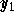.

Letbe a non-zero solution of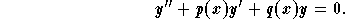Then, a second solution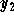independent ofcan be found as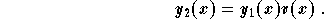Easy calculations give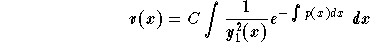,

where C is an arbitrary non-zero constant. Since we are looking for a second solution one may take C=1, to get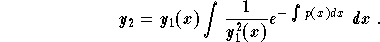Remember that this formula saves time. But, if you forget it you will have to plug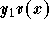into the equation to determine v(x) which may lead to mistakes !
The general solution is then given by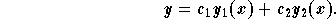Example: Find the general solution to the Legendre equation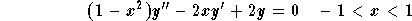,

using the fact that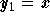is a solution.

Solution: It is easy to check that indeedis a solution. First, we need to rewrite the equation in the explicit form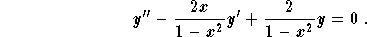We may try to find a second solution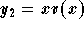by plugging it into the equation. We leave it to the reader to do that! Instead let us use the formula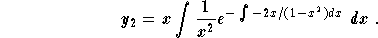Techniques of integration (of rational functions) give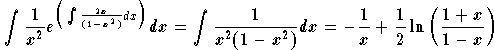,

which gives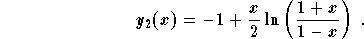The general solution is then given by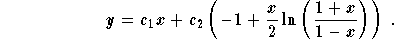Remark: The formula givingcan be obtained by also using the properties of the Wronskian (see also the discussion on the Wronskian).[Differential Equations] [First Order D.E.] [Second Order D.E.]
[Geometry] [Algebra] [Trigonometry ]
[Calculus] [Complex Variables] [Matrix Algebra]S.O.S MATHematics home page

Do you need more help? Please post your question on our S.O.S. Mathematics CyberBoard.Author: Mohamed Amine Khamsi